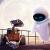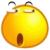## sql查询难题，对行数据统计和列数据统计问题

create or replace view  company_view as

select c.name ,c.address,

(select sum(v.id) from vehicle v  where v.companyId = c.id) as sumvehicle,

(select sum(v.ton) from vehicle v  where v.companyId = c.id) as sumvehicleton

from company c

left join vehicle  v on v.companyid = c.id ;

create or replace view  company_view as

select c.name ,c.address,sumvehicle ,sumvehicleton

from company c,(select v.id as id,sum(v.id) as sumvehicle  ,sum(v.ton) as sumvehicleton from vehicle v group by v.id)t

where t.id=c.id；效率提高了；但如果统计字段不在列上加却是在行上统计怎么办？

1```create or replace view  company_view as

select c.name ,c.address,v_type,sumvehicle ,sumvehicleton

from company c,(select v.id as id,v.v_type as v_type, sum(v.id) as sumvehicle  ,sum(v.ton) as sumvehicleton from vehicle v group by v.id,v.v_type)t
where t.id=c.id ;```

```create or replace view  company_view as

select c.name ,c.address,v_type,sumvehicle ,sumvehicleton

from company c,(select v.id as id,v.v_type as v_type,
count(v.id) as count_vehicle_type ,
count(count(v.id)) over (partition by v.v_id) as count_vehicle ,
sum(v.ton) as sumvehicleton_type,
sum(sum(v.ton)) over (partition by v.id) as sum_vehicleton
from vehicle v group by v.id,v.v_type)t
where t.id=c.id ;```

00#### 引用来自“hulubo”的答案

```create or replace view  company_view as

select c.name ,c.address,v_type,sumvehicle ,sumvehicleton

from company c,(select v.id as id,v.v_type as v_type, sum(v.id) as sumvehicle  ,sum(v.ton) as sumvehicleton from vehicle v group by v.id,v.v_type)t
where t.id=c.id ;```

```create or replace view  company_view as

select c.name ,c.address,v_type,sumvehicle ,sumvehicleton

from company c,(select v.id as id,v.v_type as v_type,
count(v.id) as count_vehicle_type ,
count(count(v.id)) over (partition by v.v_id) as count_vehicle ,
sum(v.ton) as sumvehicleton_type,
sum(sum(v.ton)) over (partition by v.id) as sum_vehicleton
from vehicle v group by v.id,v.v_type)t
where t.id=c.id ;```

```create or replace view  company_view as

select c.name ,c.address,v_type,sumvehicle ,sumvehicleton

from company c,(select v.id as id,v.v_type as v_type,
count(v.id) as count_vehicle_type ,
sum(count(v.id)) over (partition by v.v_id) as count_vehicle ,
sum(v.ton) as sumvehicleton_type,
sum(sum(v.ton)) over (partition by v.id) as sum_vehicleton
from vehicle v group by v.id,v.v_type)t
where t.id=c.id ;```